Courses
Courses for Kids
Free study material
Free LIVE classes
More

# Maths Worksheet for Kids- Division As Repeated SubtractionLIVE
Join Vedantu’s FREE Mastercalss

## Maths Worksheet for Kids| Division As Repeated Subtraction - Download Free PDF with Solutions

Learning the concepts of division and subtraction has never been this easier. It is important for the children of Class KG-3 to learn these new skills during their formative years. Here is the ideal Division as Repeated Subtraction worksheets you can add to your study material to cement your knowledge about the topics. These worksheets will make it fun and interesting to play with numbers and perform different functions like subtraction and division easily. Refer to the worksheets and solutions to understand how the division process is possible with the help of subtraction.

The worksheets for this topic are an excellent formulation of essential concepts provided by the experts at Vedantu. The prime objective of the topic is to teach some basic mathematical operations of subtraction and division to the KG-3 children. Download the worksheets for the Repeated Subtraction Method to gain more knowledge.

Last updated date: 29th Sep 2023
Total views: 21.6k
Views today: 0.21k

## Access Worksheet for Maths KG-2 Division As Repeated Subtraction

A technique called repeated subtraction, commonly referred to as division, removes an equal amount of items from a group. With this approach, the same number is continually subtracted from a larger number, and so on, until the remainder is zero or less.

### Questions:

1. Fill in the spaces below.

16/4=____

16-4=____

___-4=___

____ - 4=___

_____ - 4=___

2. Fill in the spaces below.

10/2=_______

____ – 2= _____

_____ – 2= ____

_____ –2 = ____

_____ – 2=______

_____ – 2= _____

3. Fill in the spaces below.

6/2=_______

____ – 2= _____

_____ – 2= ____

_____ –2 = ____

4. Fill in the spaces below.

10/5=_______

____ – 5= _____

_____ – 5= ____

• How many times was 5 removed from 10?

• What will come if we divide 10 by 5?

5. Tim had 10 cars, 3 of them were lost. How many toy cars does he have now?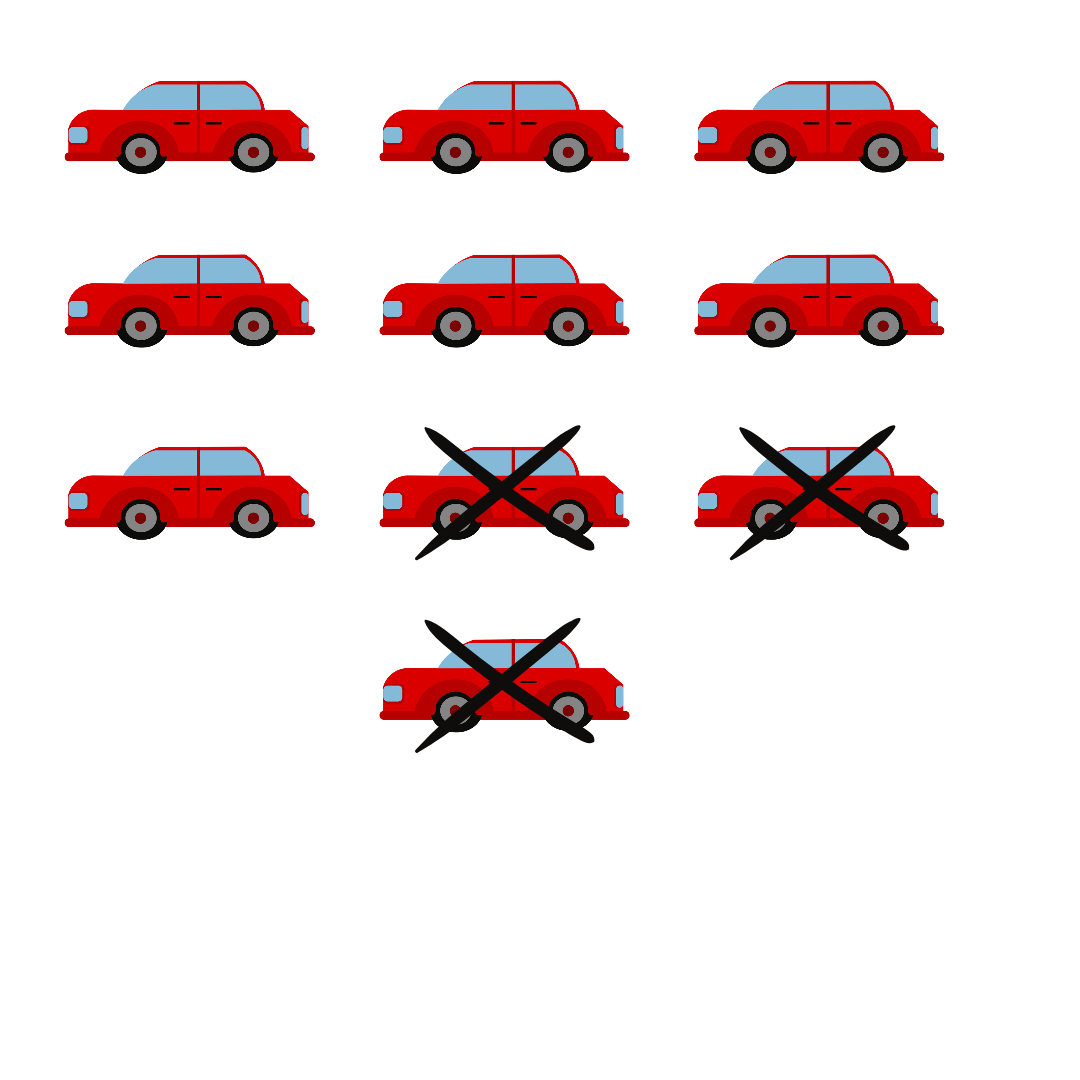10 images of toy cars and 3 are crossed

6.colour balls

Count the number of balls and divide by 5.

7. Charles had 8 marbles, and his brother took 3. How many marbles are left?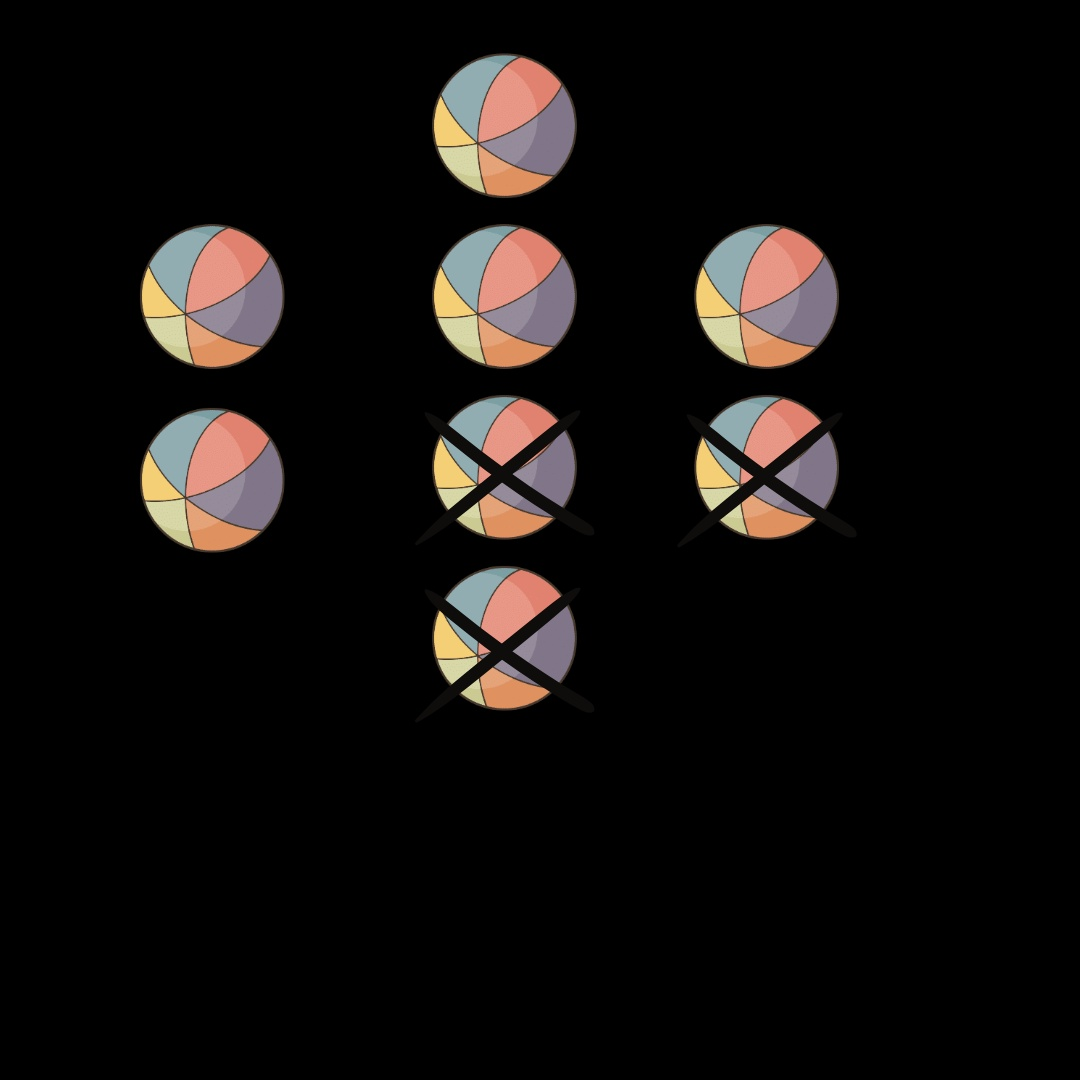8 circles 3 are crossed

8. Fill in the spaces below.

30/6=_______

____ – 6= _____

_____ – 6= ____

_____ –6 = ____

_____ – 6=______

_____ – 6= _____

9.children playing with the ball Count the number of balls.

10. Fill in the spaces below.

28/7=_______

____ – 7= _____

_____ – 7= ____

_____ –7= ____

_____ – 7=______

11. Fill in the spaces below.

20/4=_______

____ – 4= _____

_____ – 4= ____

_____ –4 = ____

_____ – 4=______

_____ – 4= _____

• How many times was 4 removed from 20?

• And what will come if we divide 20 by 4?

12. Fill in the spaces below.

15/3=_______

____ – 3= _____

_____ – 3= ____

_____ –3= ____

_____ – 3=______

_____ – 3= _____

• How many times was 3 removed from 15?

• And what will come if we divide 15 by 3?

13. Fill in the spaces below.

9/3=_______

____ – 3= _____

_____ – 3= ____

_____ –3 = ____

• How many times was 3 removed from 9?

• And what will come if we divide 9 by 3?

14. Fill in the spaces below.

8/2=_______

____ – 2= _____

_____ – 2= ____

_____ –2 = ____

_____ – 2=______

_____ – 2= _____

• How many times was 2 removed from 8?

• And what will come if we divide 8 by 2?

15.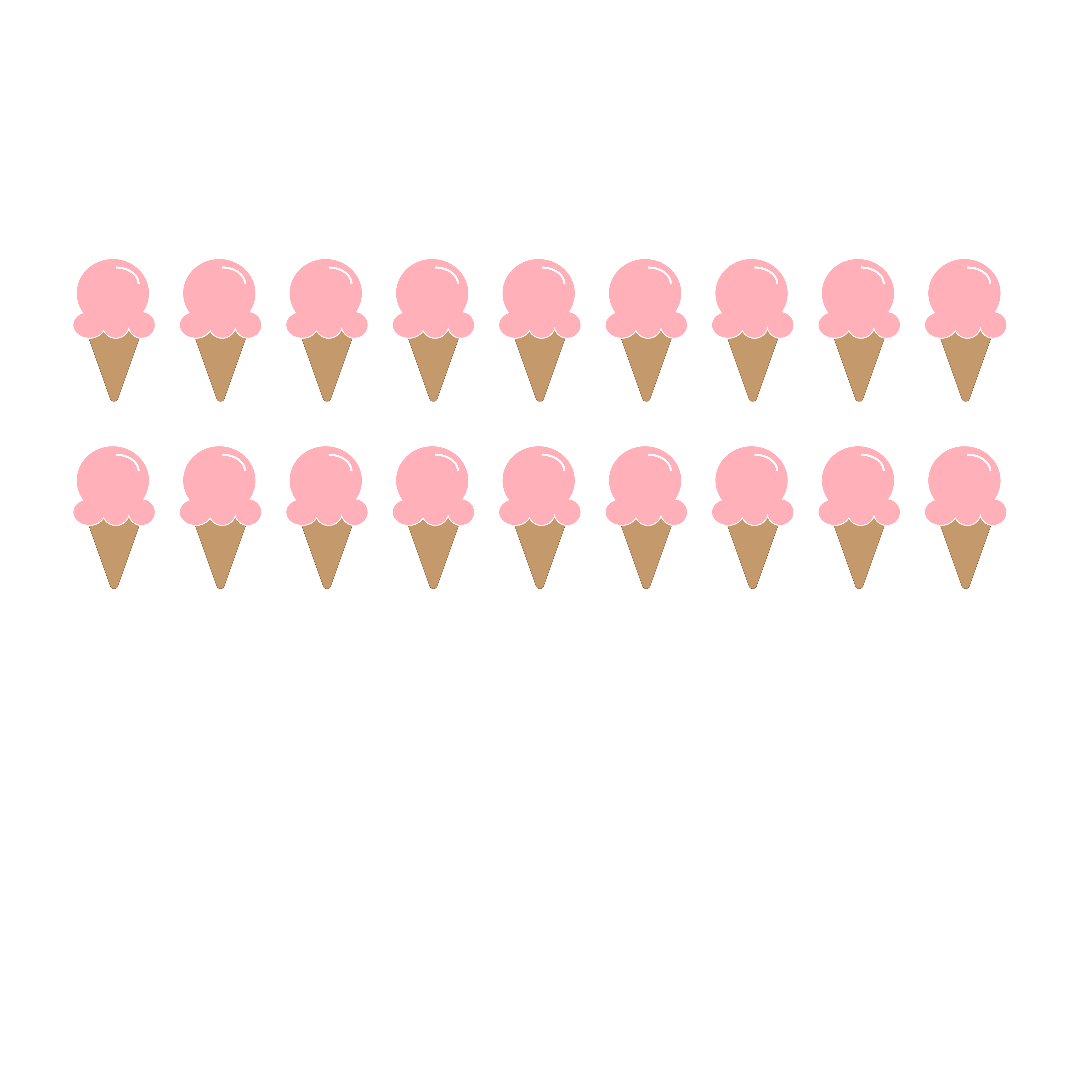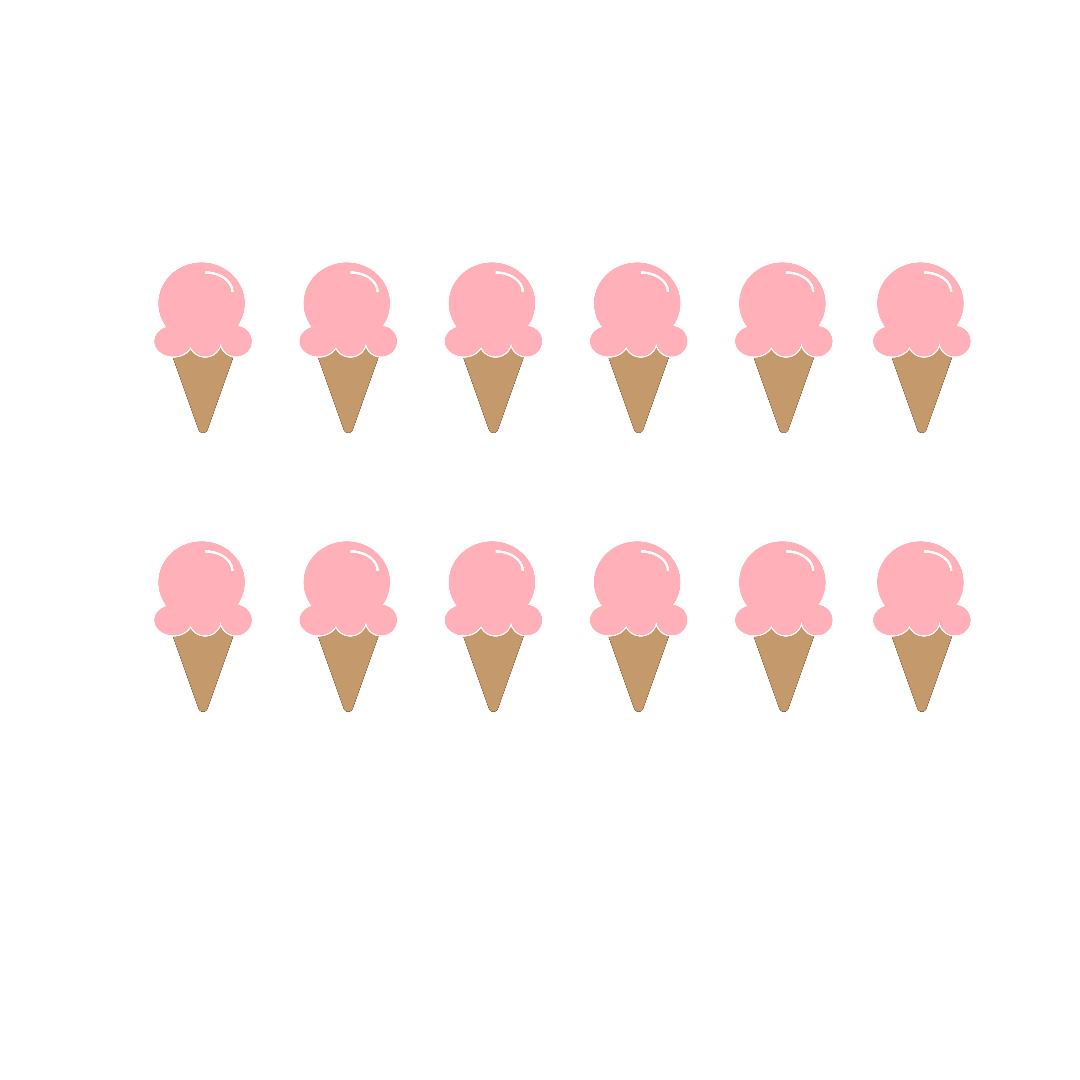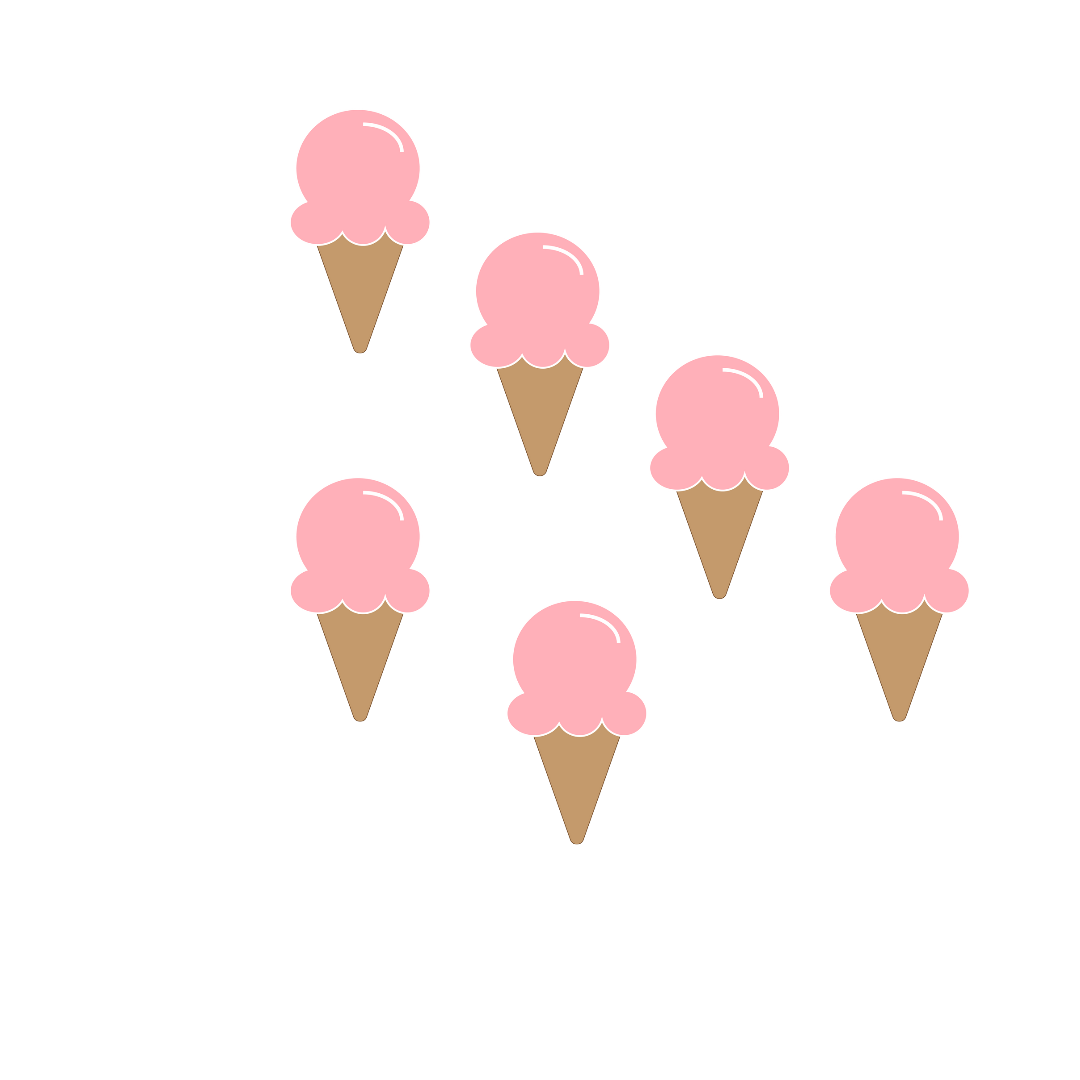ice-creams

1st- 18

2nd-12

3rd- 6

Count the total number of ice creams and divide by 3.

1.

16/4=4

16-4=12

12-4=8

8 - 4=4

4 - 4=0

2.

10/2=5

10– 2= 8

8– 2= 6

6–2 = 4

4– 2=2

2– 2= 2

3.

6/2=3

6– 2= 4

4– 2= 2

2–2 = 0

4.

10/5=2

10– 5= 5

5– 5= 0

2 times 5 was removed from 10

2 will come if we divide 10 by 5.

5.

The number of toys left is 10-3=7.

6.

The number of balls is 20, and when divided by 5 will be 20/5=4.

7.

8-3 is 5 The answer is 5 marbles.

8.

30/6=5

30– 6= 24

24– 6= 18

18–6 = 12

12– 6=6

6– 6= 0

9.

The number of balls is 20.

10.

28/7=4

28– 7= 21

21–7 = 14

14– 7=7

7– 7= 0

11.

20/4=5

20– 4= 16

16 – 4= 12

12– 4=8

8– 4= 4

4–4=0

5 times 4 was removed from 20.

5 will come if we divide 20 by 4.

12.

15/3=5

15– 3= 12

12–3 =9

9– 3=6

6– 3= 3

3-3=0

5 times 3 was removed from 15.

5 will come if we divide 15 by 3.

13.

9/3=3

9– 3=6

6– 3= 3

3-3=0

For 3 times 3 was removed from 9.

will come if we divide 9 by 3.

14.

8/2=4

8– 2= 6

6–2 = 4

4– 2=2

2– 2= 2

4 times 2 was removed from 8.

4 will come if we divide 8 by 2.

15.

The total number of ice creams is 18. 18/3=6.

## Significance of KG-3 Maths Repeated Subtraction Method Worksheets

• The Repeated Subtraction Method is a very important topic that will prepare a proper and strong foundation of mathematical operations among the students.

• The worksheets are designed by experts in the form of different questions and puzzles the students have to solve. Every single question is unique and has the potential to move the brain cells of young children.

• They can use the basic concepts of subtraction to solve these questions. Once they completely understand the topic, they will also be able to implement the methods to solve the challenging questions.

• This topic will prepare the children of KG-3 to think a bit differently about solving the questions of division. Rather than traditionally approaching the question, students will learn to apply the subtraction method to come up with the right solution.

### Benefits of Repeated Subtraction Worksheets

• The Maths experts at Vedantu have formulated the Repeated Subtraction Worksheets for the students. They have invested a lot of time, care, and effort into creating the questions that will rack up the brain cells of the children.

• There are solutions provided to the questions in the worksheet. With the help of these accurate solutions, students will learn the correct way to approach the questions and formulate the right answers on their own.

• You will find the worksheets ideal study materials to grasp a good understanding of the topic. This will also help you prepare the topic for your exams in the best way.

• The worksheets will enable students to think outside the box and move on from the traditional methods of solving a question about division. They will learn how to solve questions using the subtraction method.

If you want to know what is Repeated Subtraction Method and how it can be used, download the worksheets from the same topic right now. Vedantu is offering the worksheets for free to help the students get the content easily and effectively.

## FAQs on Maths Worksheet for Kids- Division As Repeated Subtraction

1. What is the repeated subtraction method?

The repeated subtraction method is one of the most popular methods used for dividing small and large numbers. In this method, you have to subtract the divisor from the dividend again and again till you reach 0 as a result. The number of times the divisor subtracted from the dividend is the quotient or the final answer.

2. How can you divide the number 8 from the number 2 using repeated subtraction?

You have to subtract 2 from 8 for four times to reach 0. So, the answer to this question is 4.

3. Can I answer questions easily after solving the repeated subtraction worksheets?

Yes, the explanations for all the questions have been provided in detail. You will easily answer the questions by practising the worksheets regularly.